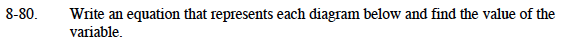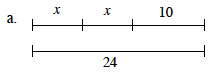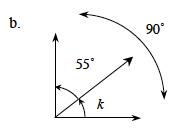Home > MC1 > Chapter 8 > Lesson 8.3.1 > Problem8-80

8-80.How are the two lines related?

Because the two lines are equal, you can write the equation:
x + x + 10 = 24

Combine like terms
2x + 10 = 24

Then solve for x:
2x = 14
x = 7

x = 7Use the same method as part (a).